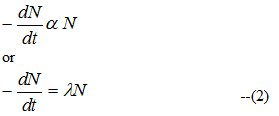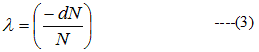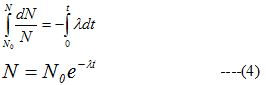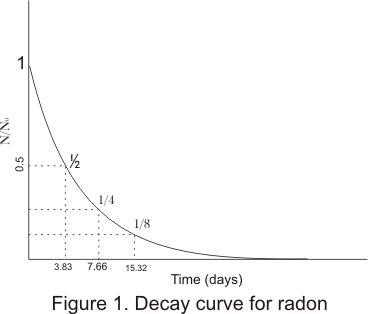• Radioactivity is a nuclear phenomenon
• When a nucleus disintegrates by emitting a particle ( α and β) or by capturing an electron from the atomic shell( K-shell) ,the process is called radioactive decay. This decay process is spontaneous.
• Let us take a radioactive sample containing N0 at time t=0 i.e, at the beginning. We wish to calculate the number N of these nuclei left after time t.
• The number of nuclei of a given radioactive sample disintegrating per sec is called the activity of that sample is
dN/dt=rate of decrease of nuclei with time=Activity of sample at time t                              --(1)
• Experimentally it is found that the activity at any instant of time t is directly proportional to the number N of parent type nuclei present at that timeWhere λ > 0 is proportionality constant and negative sign indicates that N decreases as t increases
• From equation (2) we geti.e. ,λ is fractional change in N per sec
=> λ is not merely a proportionality constant ,but it gives us the probability of decay per unit interval of time
• Hence λ is called the probability constant or decay constant or disintegration constant
• dN is the no of parent nuclei that decay between t and t+dt and we have taken N as continuous variable
• From (2)N0=No of radioactive nuclei at t=0
• From (4) we see that law of radioactive decay is exponential in character• From figure it can be noted that only half the amount of radon present initially after 3.83 days and 1/4 after 7.66 days and so on
• Plot shows that in a fixed time interval a fixed fraction of the amount of radioactive substance at the beginning of interval decays
• This faction is independent of the amount of radioactive substance and depends only on the interval of the time
• The decay constant λ is a characteristics of radioactive substance and it depends in no way on the amount of the substance present